Next: Solution of Inhomogeneous Helmholtz Up: Multipole Expansion Previous: Multipole Expansion of Vector

# Properties of Multipole Fields

Let us examine some of the properties of the multipole fields (1470)-(1471) and (1474)-(1475). Consider, first of all, the so-called near zone, in which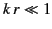. In this region,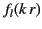is dominated by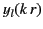, which blows up as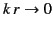, and which has the asymptotic expansion (1429), unless the coefficient ofvanishes identically. Excluding this possibility, the limiting behavior of the magnetic field for an electric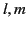multipole is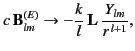(1483)

where the proportionality constant is chosen for later convenience. To find the corresponding electric field, we must take the curl of the right-hand side of the above equation. The following operator identity is useful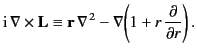(1484)

The electric field (1475) can be written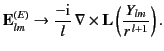(1485)

Because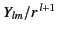is a solution of Laplace's equation, it is annihilated by the first term on the right-hand side of (1486). Consequently, for an electricmultipole, the electric field in the near zone becomes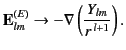(1486)

This, of course, is an electrostatic multipole field. Such a field can be obtained in a more straightforward manner by observing that, where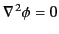, in the near zone. Solving Laplace's equation by separation of variables in spherical coordinates, and demanding that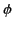be well behaved as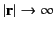, yields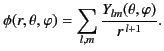(1487)

Note that (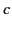times) the magnetic field (1485) is smaller than the electric field (1488) by a factor of order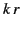. Thus, in the near zone, (times) the magnetic field associated with an electric multipole is much smaller than the corresponding electric field. For magnetic multipole fields, it is evident from Equations (1470)-(1471) and (1474)-(1475) that the roles of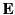and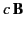are interchanged according to the transformation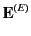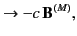(1488)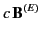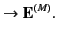(1489)

In the so-called far zone, or radiation zone, in which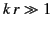, the multipole fields depend on the boundary conditions imposed at infinity. For definiteness, let us consider the case of outgoing waves at infinity, which is appropriate to radiation by a localized source. For this case, the radial functioncontains only the spherical Hankel function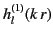. From the asymptotic form (1432), it is clear that in the radiation zone the magnetic field of an electricmultipole varies as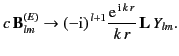(1490)

Using Equation (1475), the corresponding electric field can be written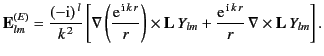(1491)

Neglecting terms that fall off faster than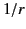, the above expression reduces to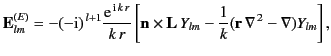(1492)

where use has been made of the identity (1486), and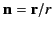is a unit vector pointing in the radial direction. The second term in square brackets is smaller than the first term by a factor of order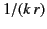, and can, therefore, be neglected in the limit. Thus, we find that the electric field in the radiation zone takes the form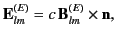(1493)

where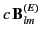is given by Equation (1492). These fields are typical radiation fields: that is, they are transverse to the radius vector, mutually orthogonal, fall off like, and are such that. To obtain expansions for magnetic multipoles, we merely make the transformation (1490)-(1491).

Consider a linear superposition of electricmultipoles with different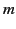values that all possess a common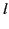value. Suppose that all multipoles correspond to outgoing waves at infinity. It follows from Equations (1474)-(1476) that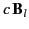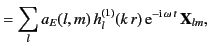(1494)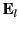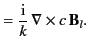(1495)

For harmonically varying fields, the time-averaged energy density is given by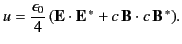(1496)

In the radiation zone, the two terms on the right-hand side of the above equation are equal. It follows that the energy contained in a spherical shell lying between radii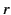and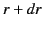is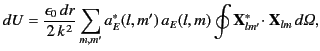(1497)

where use has been made of the asymptotic form (1432) of the spherical Hankel function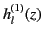. The orthogonality relation (1477) leads to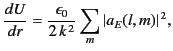(1498)

which is clearly independent of the radius. For a general superposition of electric and magnetic multipoles, the sum overbecomes a sum overand, whereas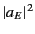becomes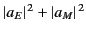. Thus, the net energy in a spherical shell situated in the radiation zone is an incoherent sum over all multipoles.

The time-averaged angular momentum density of harmonically varying electromagnetic fields is given by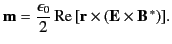(1499)

For a superposition of electric multipoles, the triple product can be expanded, and the electric field (1497) substituted, to give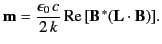(1500)

Thus, the net angular momentum contained in a spherical shell lying between radiiand(in the radiation zone) is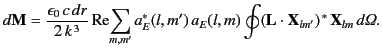(1501)

It follows from Equations (1436) and (1476) that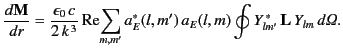(1502)

According to Equations (1439)-(1441), the Cartesian components of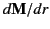can be written: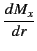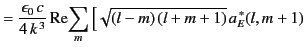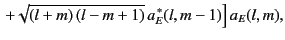(1503)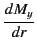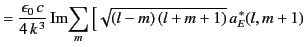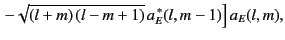(1504)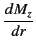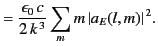(1505)

Thus, for a generalth order electric multipole that consists of a superposition of differentvalues, only the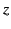component of the angular momentum takes a relatively simple form.Next: Solution of Inhomogeneous Helmholtz Up: Multipole Expansion Previous: Multipole Expansion of Vector
Richard Fitzpatrick 2014-06-27